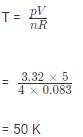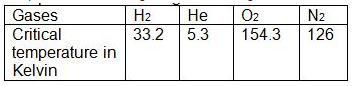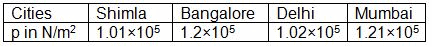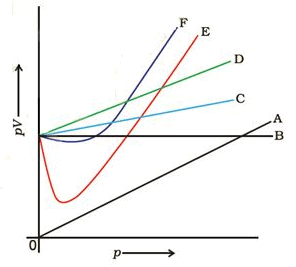Courses

# Test: States of Matter: Gases & Liquids- 1

## 25 Questions MCQ Test Chemistry Class 11 | Test: States of Matter: Gases & Liquids- 1

Description
This mock test of Test: States of Matter: Gases & Liquids- 1 for JEE helps you for every JEE entrance exam. This contains 25 Multiple Choice Questions for JEE Test: States of Matter: Gases & Liquids- 1 (mcq) to study with solutions a complete question bank. The solved questions answers in this Test: States of Matter: Gases & Liquids- 1 quiz give you a good mix of easy questions and tough questions. JEE students definitely take this Test: States of Matter: Gases & Liquids- 1 exercise for a better result in the exam. You can find other Test: States of Matter: Gases & Liquids- 1 extra questions, long questions & short questions for JEE on EduRev as well by searching above.
QUESTION: 1

### The average Kinetic energy and Thermal energy are proportional to the:

Solution:
• The average KE and Thermal energy are proportional to absolute temperature.
• When a substance is heated, some of the absorbed energy is stored within the particles, while some of the energy increases the motion of the particles. This is registered as an increase in the temperature of the substance.
QUESTION: 2

### Above the Critical temperature (TC) of carbon dioxide:

Solution:

Tc is the highest Temp where a gas can exist in liquid state. Above this no liquid state exist

QUESTION: 3

### A student forgot to add the reaction mixture to the round bottomed flask at 27 °C but instead he/she placed the flask on the flame. After a lapse of time, he realized his mistake, and using a pyrometer he found the temperature of the flask was 477 °C. What fraction of air would have been expelled out?

Solution:

Let the volume of  air in the  flask at 27° C be V1 & that of the same amount of the gas at 477° C be V2.
According to charle’s law
V2/T2  =  V1/ T1   …………………(1)
Volume of gas expelled out = V2 – V1,
Then fraction of the gas expelled out = (V2 – V1 ) / V2 = 1- (V1/ V2)     …………….(2)
Also from equation (1)  V1/ V2 = T1/ T2   …………..(3)
Substituting the values of (3) in (2), we get
Fraction of the air expelled  = 1- T1/ T2 = (T2 – T1)/ T2
= 750- 300 /750 = 0.6
Hence, fraction of air expelled out is 0.6 or 3/5

QUESTION: 4

The pressure of a 1:4 mixture of dihydrogen and dioxygen enclosed in a vessel is one atmosphere. What would be the partial pressure of dioxygen?

Solution:

PH2+PO2 , PH2=1 atm and PH2 V=(1/2)RT While PO2V=(4/32)RT

QUESTION: 5

The ease with which the electron cloud of a particle can be distorted is called its

Solution:

The polarizability in isotropic media is defined as the ratio of the induced dipole moment of an atom to the electric field that produces this dipole moment.

QUESTION: 6

Gases have much lower density than the solids and liquids because

Solution:

In gases thermal energy overcomes the the attractive intermolecular forces.

QUESTION: 7

The three states of matter of H2O are in equilibrium at

Solution:

Triple point is the point where all the three states coexist in equilibrium.

QUESTION: 8

Calculate the temperature of 4.0 mol of a gas occupying 5 dmat 3.32 bar. (R = 0.083 bar dm3K−1mol−1)

Solution:

PV=nRTQUESTION: 9

As the temperature increases, average kinetic energy of molecules increases. What would be the effect of increase of temperature on pressure provided the volume is constant?

Solution:

P α T at constant volume.

QUESTION: 10

Molecules in the interior of liquid experience intermolecular

Solution:

Net force on the molecules present inside in bulk is zero.

QUESTION: 11

Charles’ law states that pressure remaining constant, the volume of a fixed mass of a gas is

Solution:

P α T (at constant V).

QUESTION: 12

Surface tension decreases as the temperature is raised because

Solution:

Surface tension decreases as the temperature is raised because Increase in temperature increases the kinetic energy of the molecules and effectiveness of intermolecular attraction decreases.

QUESTION: 13

Calculate the total number of electrons present in 1.4 g of dinitrogen gas.

Solution:

moles of N2 =1.4/28. And 1 mole of N2= 6.022x1023 molecules of N2 And 1 molecule of N2 has 14 electrons

QUESTION: 14

Gases possess characteristic critical temperature which depends upon the magnitude of intermolecular forces between the particles. Following are the critical temperatures of some gases. From the data what would be the order of liquefaction of these gases? Start writing the order from the gas liquefying firstSolution:

Tc=8a/27Rb. As Tc increases ‘a’ increases means force of attraction between molecules also increase hence liquification increases.

QUESTION: 15

Surface tension results because

Solution:

Surface tension results because a liquid tends to minimize the number of molecules at the surface

QUESTION: 16

Gay Lussac’s law states that at constant volume, pressure of a fixed amount of a gas

Solution:

P α T (at constant V).​

QUESTION: 17

Viscous force is

Solution:

Viscous force is directly proportional to area and velocity gradient.

QUESTION: 18

The van der Waals Equation adjusts the measured volume

Solution:

nb is subtracted

QUESTION: 19

Domestic power supply in India is

Solution:

Domestic power supply in India is 230 V 50 Hz

QUESTION: 20

Capillarity results from a competition between the

Solution:

It results because intermolecular forces within the liquid (cohesive forces) and those between the liquid and the tube walls (adhesive forces)

QUESTION: 21

The statements for laws of chemical combinations are given below. Mark the statement which is not correct.

Solution:

Law of Conservation of Mass states that matter can neither be created nor destroyed. The total mass, that is, the sum of the mass of the reacting mixture and the products formed remains constant.
Law of Definite Proportions states that the proportion of elements by weight in a given compound will always remain exactly the same.
Gay Lussac’s Law of Gaseous Volume states that in a gaseous system, when the reactants combine together they always do so in volumes which bear a simple ratio to one another and to the volume of the products, under similar conditions of temperature and pressure.
Avogadro’s Law states that under the same conditions of temperature and pressure, the equal volume of all the gases contains an equal number of molecules.
Thus statement C is for Law of definite proportion
option C is correct

QUESTION: 22

Water has high surface tension and high capillarity because of

Solution:

Water molecules form extensive Hydrogen Bonds.

QUESTION: 23

The van der Waals Equation adjusts the measured pressure

Solution:

an2/V2 is added.

QUESTION: 24

Atmospheric pressures recorded in different cities are as follows: Consider the above data and mark the place at which liquid will boil first.Solution:

Shimla has lowest atmospheric pressure, hence liquidwill boil first in this city. Boiling of a liquid takes place, when the vapour pressure becomes equal to the atmospheric pressure.s.

QUESTION: 25

Which curve in Figure represents the curve of ideal gas?Solution:

for ideal gas PV=constant.